xInterpretation (logic)Encyclopedia
An interpretation is an assignment of meaning to the symbols
Symbol (formal)
For other uses see Symbol In logic, symbols build literal utility to illustrate ideas. A symbol is an abstraction, tokens of which may be marks or a configuration of marks which form a particular pattern...

of a formal language
Formal language
A formal language is a set of words—that is, finite strings of letters, symbols, or tokens that are defined in the language. The set from which these letters are taken is the alphabet over which the language is defined. A formal language is often defined by means of a formal grammar...

. Many formal languages used in mathematics
Mathematics
Mathematics is the study of quantity, space, structure, and change. Mathematicians seek out patterns and formulate new conjectures. Mathematicians resolve the truth or falsity of conjectures by mathematical proofs, which are arguments sufficient to convince other mathematicians of their validity...

, logic
Logic
In philosophy, Logic is the formal systematic study of the principles of valid inference and correct reasoning. Logic is used in most intellectual activities, but is studied primarily in the disciplines of philosophy, mathematics, semantics, and computer science...

, and theoretical computer science
Theoretical computer science
Theoretical computer science is a division or subset of general computer science and mathematics which focuses on more abstract or mathematical aspects of computing....

are defined in solely syntactic
Syntax
In linguistics, syntax is the study of the principles and rules for constructing phrases and sentences in natural languages....

terms, and as such do not have any meaning until they are given some interpretation. The general study of interpretations of formal languages is called formal semantics.

The most commonly studied formal logics are propositional logic, predicate logic
Predicate logic
In mathematical logic, predicate logic is the generic term for symbolic formal systems like first-order logic, second-order logic, many-sorted logic or infinitary logic. This formal system is distinguished from other systems in that its formulae contain variables which can be quantified...

and their modal analogs, and for these there are standard ways of presenting an interpretation. In these contexts an interpretation is a function
Function (mathematics)
In mathematics, a function associates one quantity, the argument of the function, also known as the input, with another quantity, the value of the function, also known as the output. A function assigns exactly one output to each input. The argument and the value may be real numbers, but they can...

that provides the extension
Extension (predicate logic)
The extension of a predicatea truth-valued functionis the set of tuples of values that, used as arguments, satisfy the predicate. Such a set of tuples is a relation.For example the statement "d2 is the weekday following d1"...

of symbols and strings of symbols of an object language. For example, an interpretation function could take the predicate T (for "tall") and assign it the extension {a} (for "Abraham Lincoln"). Note that all our interpretation does is assign the extension {a} to the non-logical constant T, and does not make a claim about whether T is to stand for tall and 'a' for Abraham Lincoln. Nor does logical interpretation have anything to say about logical connectives like 'and', 'or' and 'not'. Though we may take these symbols to stand for certain things or concepts, this is not determined by the interpretation function.

An interpretation often (but not always) provides a way to determine the truth values of sentences
Sentence (mathematical logic)
In mathematical logic, a sentence of a predicate logic is a boolean-valued well formed formula with no free variables. A sentence can be viewed as expressing a proposition, something that may be true or false...

in a language. If a given interpretation assigns the value True to a sentence or theory
Theory (mathematical logic)
In mathematical logic, a theory is a set of sentences in a formal language. Usually a deductive system is understood from context. An element \phi\in T of a theory T is then called an axiom of the theory, and any sentence that follows from the axioms is called a theorem of the theory. Every axiom...

, the interpretation is called a model of that sentence or theory.

## Formal languages

A formal language consists of a fixed collection of sentences (also called words or formulas, depending on the context) composed from a fixed set of letters or symbols. The inventory from which these letters are taken is called the alphabet over which the language is defined. The essential feature of a formal language is that its syntax can be defined without reference to interpretation. We can determine that (P or Q) is a well-formed formula even without knowing whether it is true or false.

To distinguish the strings of symbols that are in a formal language from arbitrary strings of symbols, the former are sometimes called well-formed formulæ (wffs)

### Example

A formal language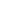is defined with the
alphabet α = {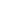,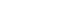}. A word is declared to be inif it begins withand is composed solely of the symbolsand.

A possible interpretation ofwould assign the decimal digit '1' toand '0' to. Thereforewould denote 101 under this interpretation of.

### Logical constants

In the specific cases of propositional logic and predicate logic, the formal languages considered have alphabets that are divided into two sets: the logical symbols (logical constants) and the non-logical symbols. The idea behind this terminology is that logical symbols have the same meaning regardless of the subject matter being studied, while non-logical symbols change in meaning depending on the area of investigation.

Logical constants are always given the same meaning by every interpretation of the standard kind, so that only the meanings of the non-logical symbols are changed. Logical constants include quantifier symbols ∀ and ∃, symbols for logical connective
Logical connective
In logic, a logical connective is a symbol or word used to connect two or more sentences in a grammatically valid way, such that the compound sentence produced has a truth value dependent on the respective truth values of the original sentences.Each logical connective can be expressed as a...

s, parentheses and other grouping symbols, and (in many treatments) the equality symbol =.

## General properties of truth-functional interpretations

Many of the commonly studied interpretations associate each sentence in a formal language with a single truth value, either True or False. These interpretations are called truth functional; they include the usual interpretations of propositional and first-order logic. The sentences that are made true by a particular assignment are said to be satisfied by that assignment.

No sentence can be made both true and false by the same interpretation, but it is possible that the truth value of the same sentence can be different under different interpretations. A sentence is consistent
Consistency
Consistency can refer to:* Consistency , the psychological need to be consistent with prior acts and statements* "Consistency", an 1887 speech by Mark Twain...

if it is true under at least one interpretation; otherwise it is inconsistent. A sentence φ is said to be logically valid if it is satisfied by every interpretation (if φ is satisfied by every interpretation that satisfies ψ then φ is said to be a logical consequence of ψ).

### Logical connectives

Some of the logical symbols of a language (other than quantifiers) are truth-functional connectives
Logical connective
In logic, a logical connective is a symbol or word used to connect two or more sentences in a grammatically valid way, such that the compound sentence produced has a truth value dependent on the respective truth values of the original sentences.Each logical connective can be expressed as a...

that represent truth functions - functions that take truth values as arguments and return truth values as outputs (in other words, these are operations on truth values of sentences).

The truth-functional connectives enable compound sentences to be built up from simpler sentences. In this way, the truth value of the compound sentence is defined as a certain truth function of the truth values of the simpler sentences. The connectives are usually taken to be logical constants, meaning that the meaning of the connectives is always the same, independent of what interpretations are given to the other symbols in a formula.

This is how we define logical connectives in propositional logic:
• ~Φ is True iff Φ is False.
• (Φ Ψ) is True iff Φ is True and Ψ is True.
• (Φ Ψ) is True iff (Φ Ψ) is True.
• (Φ Ψ) is True iff (Φ is True Ψ is True).
• (Φ Ψ) is True iff (Φ Ψ) is True and (Ψ Φ) is True.

So under a given interpretation of all the sentence letters Φ and Ψ (i.e., after assigning a truth-value to each sentence letter), we can determine the truth-values of all formulas that have them as constituents, as a function of the logical connectives. The following table shows how this kind of thing looks. The first two columns show the truth-values of the sentence letters as determined by the four possible interpretations. The other columns show the truth-values of formulas built off of these sentence letters, with truth-values determined recursively.

Logical connectives
Interpretation Φ Ψ Φ (Φ Ψ) (Φ Ψ) (Φ Ψ) (Φ Ψ)
#1 T T F T T T T
#2 T F F F T F F
#3 F T T F T T F
#4 F F T F F T T

Now its easier to see what makes a formula logically valid. Take the formula F: (Φ ~Φ). If our interpretation function makes Φ True, then ~Φ is made False by the negation connective. Since the disjunct Φ of F is True under that interpretation, F is True. Now the only other possible interpretation of Φ makes it False, and if so, ~Φ is made True by the negation function. That would make F True again, since one of Fs disjuncts, ~Φ, would be true under this interpretation. Since these two interpretations for F are the only possible logical interpretations, and since F comes out True for both, we say that it is logically valid or tautologous.

## Interpretation of a theory

An interpretation of a theory is the relationship between a theory and some subject matter when there is a many-to-one correspondence between certain elementary statements of the theory, and certain statements related to the subject matter. If every elementary statement in the theory has a correspondent it is called a full interpretation, otherwise it is called a partial interpretation.

## Interpretations for propositional logic

The formal language for propositional logic consists of formulas built up from propositional symbols (also called sentential symbols, sentential variables, and propositional variables) and logical connectives. The only non-logical symbol
Non-logical symbol
In logic, the formal languages used to create expressions consist of symbols which can be broadly divided into constants and variables. The constants of a language can further be divided into logical symbols and non-logical symbols .The non-logical symbols of a language of first-order logic consist...

s in a formal language for propositional logic are the propositional symbols, which are often denoted by capital letters. To make the formal language precise, a specific set of propositional symbols must be fixed.

The standard kind of interpretation in this setting is a function that maps each propositional symbol to one of the truth values true and false. This function is known as a truth assignment or valuation function. In many presentations, it is literally a truth value that is assigned, but some presentations assign truthbearer
Truthbearer
Truth-bearer is a term used to designate entities that are either true or false and nothing else. The thesis that some things are true while others are false raises the question of the nature of these things. Since there is divergence of opinion on the matter, the term truthbearer is used to be...

For a language with n distinct propositional variables there are 2n distinct possible interpretations. For any particular variable a, for example, there are 21=2 possible interpretations: 1) a is assigned T, or 2) a is assigned F. For the pair a, b there are 22=4 possible interpretations: 1) both are assigned T, 2) both are assigned F, 3) a is assigned T and b is assigned F, or 4) a is assigned F and b is assigned T.

Given any truth assignment for a set of propositional symbols, there is a unique extension to an interpretation for all the propositional formulas built up from those variables. This extended interpretation is defined inductively, using the truth-table definitions of the logical connectives discussed above.

## First-order logic

Unlike propositional logic, where every language is the same apart from a choice of a different set of propositional variables, there are many different first-order languages. Each first-order language is defined by a signature. The signature consists of a set of non-logical symbols and an identification of each of these symbols as a constant symbol, a function symbol, or a predicate symbol. In the case of function and predicate symbols, a natural number arity
Arity
In logic, mathematics, and computer science, the arity of a function or operation is the number of arguments or operands that the function takes. The arity of a relation is the dimension of the domain in the corresponding Cartesian product...

is also assigned. The alphabet for the formal language consists of logical constants, the equality relation symbol =, all the symbols from the signature, and an additional infinite set of symbols known as variables.

For example, in the language of rings
Ring (mathematics)
In mathematics, a ring is an algebraic structure consisting of a set together with two binary operations usually called addition and multiplication, where the set is an abelian group under addition and a semigroup under multiplication such that multiplication distributes over addition...

, there are constant symbols 0 and 1, two binary function symbols + and ·, and no binary relation symbols. (Here the equality relation is taken as a logical constant.)

Again, we might define a first-order language L, as consisting of individual symbols a, b, and c; predicate symbols F,G, H, I and J; variables x,y,z; no function letters; no sentential symbols.

### Formal languages for first-order logic

Given a signature σ, the corresponding formal language is known as the set of σ-formulas. Each σ-formula is built up out of atomic formulas by means of logical connectives; atomic formulas are built from terms using predicate symbols. The formal definition of the set of σ-formulas proceeds in the other direction: first, terms are assembled from the constant and function symbols together with the variables. Then, terms can be combined into an atomic formula using a predicate symbol (relation symbol) from the signature or the special predicate symbol "=" for equality (see the section "Interpreting equality" below). Finally, the formulas of the language are assembled from atomic formulas using the logical connectives and quantifiers.

### Interpretations of a first-order language

To ascribe meaning to all sentences of a first-order language, the following information is needed.
• A domain of discourse
Domain of discourse
In the formal sciences, the domain of discourse, also called the universe of discourse , is the set of entities over which certain variables of interest in some formal treatment may range...

D, usually required to be non-empty (see below).
• For every constant symbol, an element of D as its interpretation.
• For every n-ary function symbol, an n-ary function from D to D as its interpretation (that is, a function Dn → D).
• For every n-ary predicate symbol, an n-ary relation on D as its interpretation (that is, a subset of Dn).

An object carrying this information is known as a structure
Structure (mathematical logic)
In universal algebra and in model theory, a structure consists of a set along with a collection of finitary operations and relations which are defined on it....

(of signature σ, or σ-structure, or L-structure), or as a "model".

The information specified in the interpretation provides enough information to give a truth value to any atomic formula, after each of its free variables, if any, has been replaced by an element of the domain. The truth value of an arbitrary sentence is then defined inductively using the T-schema
T-schema
The T-schema or truth schema is used to give an inductive definition of truth which lies at the heart of any realisation of Alfred Tarski's semantic theory of truth...

, which is a definition of first-order semantics developed by Alfred Tarski. The T-schema interprets the logical connectives using truth tables, as discussed above. Thus, for example, is satisfied if and only if both φ and ψ are satisfied.

This leaves the issue of how to interpret formulas of the form and . The domain of discourse forms the range
Range (mathematics)
In mathematics, the range of a function refers to either the codomain or the image of the function, depending upon usage. This ambiguity is illustrated by the function f that maps real numbers to real numbers with f = x^2. Some books say that range of this function is its codomain, the set of all...

for these quantifiers. The idea is that the sentence is true under an interpretation exactly when every substitution instance of φ(x), where x is replaced by some element of the domain, is satisfied. The formula is satisfied if there is at least one element d of the domain such that φ(d) is satisfied.

Strictly speaking, a substitution instance such as the formula φ(d) mentioned above is not a formula in the original formal language of φ, because d is an element of the domain. There are two ways of handling this technical issue. The first is to pass to a larger language in which each element of the domain is named by a constant symbol. The second is to add to the interpretation a function that assigns each variable to an element of the domain. Then the T-schema can quantify over variations of the original interpretation in which this variable assignment function is changed, instead of quantifying over substitution instances.

Some authors also admit propositional variable
Propositional variable
In mathematical logic, a propositional variable is a variable which can either be true or false...

s in first-order logic, which must then also be interpreted. A propositional variable can stand on its own as an atomic formula. The interpretation of a propositional variable is one of the two truth values true and false.

Because the first-order interpretations described here are defined in set theory, they do not associate each predicate symbol with a property (or relation), but rather with the extension of that property (or relation). In other words, these first-order interpretations are extensional
Extensional definition
An extensional definition of a concept or term formulates its meaning by specifying its extension, that is, every object that falls under the definition of the concept or term in question....

not intensional
Intensional definition
In logic and mathematics, an intensional definition gives the meaning of a term by specifying all the properties required to come to that definition, that is, the necessary and sufficient conditions for belonging to the set being defined....

.

### Example of a first-order interpretation

An example of interpretation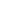of the language L described above is as follows.
• Domain: A chess set
• Individual constants: a: The white King b: The black Queen c: The white Kings's pawn
• F(x): x is a piece
• G(x): x is a pawn
• H(x): x is black
• I(x): x is white
• J(x, y): x can capture y

In the interpretationof L:
• the following are true sentences: F(a), G(c), H(b), I(a) J(b, c),
• the following are false sentences: J(a, c), G(a).

### Non-empty domain requirement

As stated above, a first-order interpretation is usually required to specify a nonempty set as the domain of discourse. The reason for this requirement is to guarantee that equivalences such as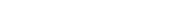,

where x is not a free variable of φ, are logically valid. This equivalence holds in every interpretation with a nonempty domain, but does not always hold when empty domains are permitted. For example, the equivalence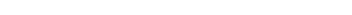fails in any structure with an empty domain. Thus the proof theory of first-order logic becomes more complicated when empty structures are permitted. However, the gain in allowing them is negligible, as both the intended interpretations and the interesting interpretations of the theories people study have non-empty domains.

Empty relations do not cause any problem for first-order interpretations, because there is no similar notion of passing a relation symbol across a logical connective, enlarging its scope in the process. Thus it is acceptable for relation symbols to be interpreted as being identically false. However, the interpretation of a function symbol must always assign a well-defined and total function to the symbol.

### Interpreting equality

The equality relation is often treated specially in first order logic and other predicate logics. There are two general approaches.

The first approach is to treat equality as no different than any other binary relation. In this case, if an equality symbol is included in the signature, it is usually necessary to add various axioms about equality to axiom systems (for example, the substitution axiom saying that if a = b and R(a) holds then R(b) holds as well). This approach to equality is most useful when studying signatures that do not include the equality relation, such as the signature for set theory
Set theory
Set theory is the branch of mathematics that studies sets, which are collections of objects. Although any type of object can be collected into a set, set theory is applied most often to objects that are relevant to mathematics...

or the signature for second-order arithmetic
Second-order arithmetic
In mathematical logic, second-order arithmetic is a collection of axiomatic systems that formalize the natural numbers and their subsets. It is an alternative to axiomatic set theory as a foundation for much, but not all, of mathematics. It was introduced by David Hilbert and Paul Bernays in their...

in which there is only an equality relation for numbers, but not an equality relation for set of numbers.

The second approach is to treat the equality relation symbol as a logical constant that must be interpreted by the real equality relation in any interpretation. An interpretation that interprets equality this way is known as a normal model, so this second approach is the same as only studying interpretations that happen to be normal models. The advantage of this approach is that the axioms related to equality are automatically satisfied by every normal model, and so they do not need to be explicitly included in first-order theories when equality is treated this way. This second approach is sometimes called first order logic with equality, but many authors adopt it for the general study of first-order logic without comment.

There are a few other reasons to restrict study of first-order logic to normal models. First, it is known that any first-order interpretation in which equality is interpreted by an equivalence relation
Equivalence relation
In mathematics, an equivalence relation is a relation that, loosely speaking, partitions a set so that every element of the set is a member of one and only one cell of the partition. Two elements of the set are considered equivalent if and only if they are elements of the same cell...

and satisfies the substitution axioms for equality can be cut down to an elementarily equivalent
Elementary substructure
In model theory, a field within mathematical logic, two structures M and N of the same signature σ are called elementarily equivalent if they satisfy the same first-order σ-sentences....

interpretation on a subset of the original domain. Thus there is little additional generality in studying non-normal models. Second, if non-normal models are considered, then every consistent theory has an infinite model; this affects the statements of results such as the Löwenheim–Skolem theorem
Löwenheim–Skolem theorem
In mathematical logic, the Löwenheim–Skolem theorem, named for Leopold Löwenheim and Thoralf Skolem, states that if a countable first-order theory has an infinite model, then for every infinite cardinal number κ it has a model of size κ...

, which are usually stated under the assumption that only normal models are considered.

### Many-sorted first-order logic

A generalization of first order logic considers languages with more than one sort of variables. The idea is different sorts of variables represent different types of objects. Every sort of variable can be quantified; thus an interpretation for a many-sorted language has a separate domain for each of the sorts of variables to range over (there is an infinite collection of variables of each of the different sorts). Function and relation symbols, in addition to having arities, are specified so that each of their arguments must come from a certain sort.

One example of many-sorted logic is for planar Euclidean geometry
Euclidean geometry
Euclidean geometry is a mathematical system attributed to the Alexandrian Greek mathematician Euclid, which he described in his textbook on geometry: the Elements. Euclid's method consists in assuming a small set of intuitively appealing axioms, and deducing many other propositions from these...

. There are two sorts; points and lines. There is an equality relation symbol for points, an equality relation symbol for lines, and a binary incidence relation E which takes one point variable and one line variable. The intended interpretation of this language has the point variables range over all points on the Euclidean plane, the line variable range over all lines on the plane, and the incidence relation E(p,l) holds if and only if point p is on line l.

## Higher-order predicate logics

A formal language for higher-order predicate logic
Higher-order logic
In mathematics and logic, a higher-order logic is a form of predicate logic that is distinguished from first-order logic by additional quantifiers and a stronger semantics...

looks much the same as a formal language for first-order logic. The difference is that there are now many different types of variables. Some variables correspond to elements of the domain, as in first-order logic. Other variables correspond to objects of higher type: subsets of the domain, functions from the domain, functions that take a subset of the domain and return a function from the domain to subsets of the domain, etc. All of these types of variables can be quantified.

There are two kinds of interpretations commonly employed for higher-order logic. Full semantics require that, once the domain of discourse is satisfied, the higher-order variables range over all possible elements of the correct type (all subsets of the domain, all functions from the domain to itself, etc.). Thus the specification of a full interpretation is the same as the specification of a first-order interpretation. Henkin semantics, which are essentially multi-sorted first-order semantics, require the interpretation to specify a separate domain for each type of higher-order variable to range over. Thus an interpretation in Henkin semantics includes a domain D, a collection of subsets of D, a collection of functions from D to D, etc. The relationship between these two semantics is an important topic in higher order logic.

## Non-classical interpretations

The interpretations of propositional logic and predicate logic described above are not the only possible interpretations. In particular, there are other types of interpretations that are used in the study of non-classical logic
Non-classical logic
Non-classical logics is the name given to formal systems which differ in a significant way from standard logical systems such as propositional and predicate logic. There are several ways in which this is done, including by way of extensions, deviations, and variations...

(such as intuitionistic logic
Intuitionistic logic
Intuitionistic logic, or constructive logic, is a symbolic logic system differing from classical logic in its definition of the meaning of a statement being true. In classical logic, all well-formed statements are assumed to be either true or false, even if we do not have a proof of either...

), and in the study of modal logic.

Interpretations used to study non-classical logic include topological models, Boolean valued models, and Kripke models. Modal logic
Modal logic
Modal logic is a type of formal logic that extends classical propositional and predicate logic to include operators expressing modality. Modals — words that express modalities — qualify a statement. For example, the statement "John is happy" might be qualified by saying that John is...

is also studied using Kripke models.

## Intended interpretations

Many formal languages are associated with a particular interpretation that is used to motivate them. For example, the first-order signature for set theory includes only one binary relation, ∈, which is intended to represent set membership, and the domain of discourse in a first-order theory of the natural numbers is intended be the set of natural numbers. However, there are always other unintended interpretations in which either the domain of discourse or the non-logical symbols are not given their intended meanings.

In the context of Peano arithmetic, the intended interpretation is called the standard model of arithmetic. It consists of the natural numbers with their ordinary arithmetical operations. All models that are isomorphic to the one just given are also called standard; these models all satisfy the Peano axioms
Peano axioms
In mathematical logic, the Peano axioms, also known as the Dedekind–Peano axioms or the Peano postulates, are a set of axioms for the natural numbers presented by the 19th century Italian mathematician Giuseppe Peano...

. There are also non-standard models of the Peano axioms, which contain elements not correlated with any natural number.

## Other concepts of interpretation

There are other uses of the term "interpretation" that are commonly used, which do not refer to the assignment of meanings to formal languages.

In model theory, a structure A is said to interpret a structure B if there is a definable subset D of A, and definable relations and functions on D, such that B is isomorphic to the structure with domain D and these functions and relations. In some settings, it is not the domain D that is used, but rather D modulo an equivalence relation definable in A. For additional information, see Interpretation (model theory)
Interpretation (model theory)
In model theory, interpretation of a structure M in another structure N is a technical notion that approximates the idea of representing M inside N. For example every reduct or definitional expansion of a structure N has an interpretation in N.Many model-theoretic properties are preserved under...

.

A theory T is said to interpret another theory S if there is a finite extension by definitions T′ of T such that S is contained in T′.

• Herbrand interpretation
Herbrand interpretation
In mathematical logic, a Herbrand interpretation is an interpretation in which all constants and function symbols are assigned very simple meanings. Specifically, every constant is interpreted as itself, and every function symbol is interpreted as the function that applies it...

• Interpretation (model theory)
Interpretation (model theory)
In model theory, interpretation of a structure M in another structure N is a technical notion that approximates the idea of representing M inside N. For example every reduct or definitional expansion of a structure N has an interpretation in N.Many model-theoretic properties are preserved under...

• Logical system
• Löwenheim-Skolem theorem
• Modal logic
Modal logic
Modal logic is a type of formal logic that extends classical propositional and predicate logic to include operators expressing modality. Modals — words that express modalities — qualify a statement. For example, the statement "John is happy" might be qualified by saying that John is...

• Model (abstract)
• Model theory
Model theory
In mathematics, model theory is the study of mathematical structures using tools from mathematical logic....

• Satisfiable